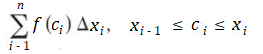# Norm of a Partition (Mesh): Definition, Formula, How to Find it

The norm of a partition (sometimes called the mesh of a partition) is the width of the longest subinterval in a Riemann integral. A “partition” is just another name for one of the segments that you create by chopping a function up into pieces when finding Riemann Sums. Riemann sums are used to approximate areas, so smaller rectangles (ideally, with widths close to zero) lead to better approximations. Therefore, the norm is often used to determine how “good” the partition is.

## Norm of a Partition in a Riemann Sum

The norm of a partition ||Δ|| is the width of the biggest subinterval in a Riemann Sum defined as follows (Larson & Edwards, 2008).

Suppose that a function f is defined on a closed interval [a, b] Also suppose that Δ is a partition of [a, b] given by

a = x0 < x1 < x2 < … < xn – 1, xn = b

Where Δxi is the width of the ith subinterval.

Then a Riemann sum of f, for the partition Δ is the sum:Where ci is any point in the ith subinterval [xi – 1, xi]

## How to find the norm of a partition

In a general sense, the norm of a partition is just the length of the largest subinterval:
max{Δ1, Δ2, …Δk, …, Δn}.

There are a couple of formulas you can use to find the norm of a partition. Which formula you use depends on if your intervals are all the same width (called regular partitions) or different sizes (called general partitions).

1. Regular partitions:
||Δ|| = Δx = (ba )/ n

Example: Let’s say your closed interval is [1, 9] and you have 4 partitions. The norm of the partition is (9 – 1) / 4 = 2

2. General partitions:
The norm for a general partition can be quantified by the following inequality:

ba / ||Δ|| ≤ n

As the number of subintervals, n, approaches infinity, the norm, ||Δ||, approaches 0. This fact was important in Riemann’s original definition of the value of an integral, which he defined as the limit of Riemann sums as the partition norms approach zero (Joyce, 2013).

## References

Joyce, D. (2013). The definition of the integral. Math 120 Calculus I. Retrieved May 14, 2020 from: https://www2.clarku.edu/faculty/djoyce/ma120/integral.pdf
Larson, R. & Edwards, B. (2008). Calculus of a Single Variable. Cengage Learning.

CITE THIS AS:
Stephanie Glen. "Norm of a Partition (Mesh): Definition, Formula, How to Find it" From StatisticsHowTo.com: Elementary Statistics for the rest of us! https://www.statisticshowto.com/norm-of-a-partition/